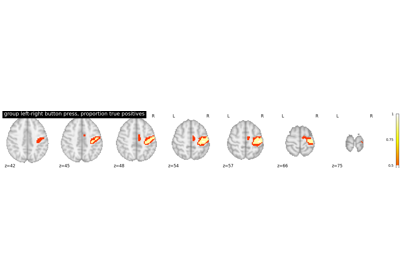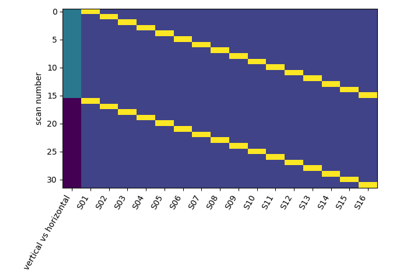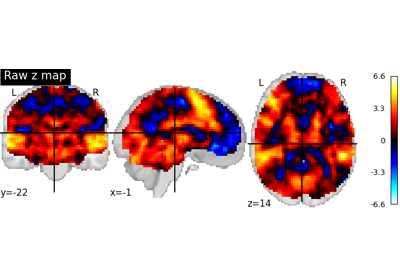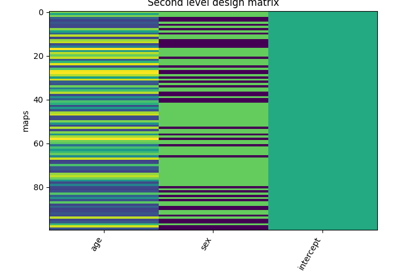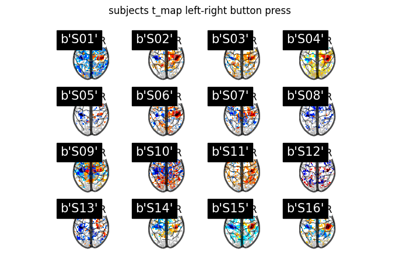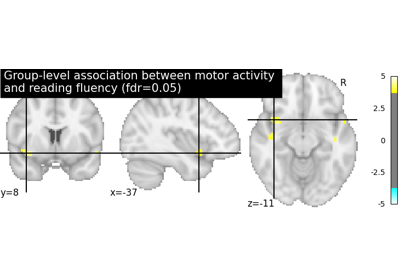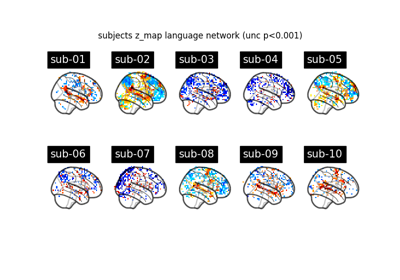Note

This page is a reference documentation. It only explains the class signature, and not how to use it. Please refer to the user guide for the big picture.

3.8.1. nistats.second_level_model.SecondLevelModel¶

class nistats.second_level_model.SecondLevelModel(mask_img=None, smoothing_fwhm=None, memory=Memory(location=None), memory_level=1, verbose=0, n_jobs=1, minimize_memory=True)

Implementation of the General Linear Model for multiple subject fMRI data

Parameters

Mask to be used on data. If an instance of masker is passed, then its mask will be used. If no mask is given, it will be computed automatically by a MultiNiftiMasker with default parameters. Automatic mask computation assumes first level imgs have already been masked.

smoothing_fwhm: float, optional

If smoothing_fwhm is not None, it gives the size in millimeters of the spatial smoothing to apply to the signal.

memory: string, optional

Path to the directory used to cache the masking process and the glm fit. By default, no caching is done. Creates instance of joblib.Memory.

memory_level: integer, optional

Rough estimator of the amount of memory used by caching. Higher value means more memory for caching.

verboseinteger, optional

Indicate the level of verbosity. By default, nothing is printed. If 0 prints nothing. If 1 prints final computation time. If 2 prints masker computation details.

n_jobsinteger, optional

The number of CPUs to use to do the computation. -1 means ‘all CPUs’, -2 ‘all CPUs but one’, and so on.

minimize_memoryboolean, optional

Gets rid of some variables on the model fit results that are not necessary for contrast computation and would only be useful for further inspection of model details. This has an important impact on memory consumption. True by default.

__init__(self, mask_img=None, smoothing_fwhm=None, memory=Memory(location=None), memory_level=1, verbose=0, n_jobs=1, minimize_memory=True)

Initialize self. See help(type(self)) for accurate signature.

compute_contrast(self, second_level_contrast=None, first_level_contrast=None, second_level_stat_type=None, output_type='z_score')

Generate different outputs corresponding to the contrasts provided e.g. z_map, t_map, effects and variance.

Parameters
second_level_contrast: str or array of shape (n_col), optional

Where n_col is the number of columns of the design matrix. The string can be a formula compatible with pandas.DataFrame.eval. Basically one can use the name of the conditions as they appear in the design matrix of the fitted model combined with operators +- and combined with numbers with operators +-*/. The default (None) is accepted if the design matrix has a single column, in which case the only possible contrast array() is applied; when the design matrix has multiple columns, an error is raised.

first_level_contrast: str or array of shape (n_col) with respect to

FirstLevelModel, optional

In case a list of FirstLevelModel was provided as second_level_input, we have to provide a contrast to apply to the first level models to get the corresponding list of images desired, that would be tested at the second level. In case a pandas DataFrame was provided as second_level_input this is the map name to extract from the pandas dataframe map_name column. It has to be a ‘t’ contrast.

second_level_stat_type: {‘t’, ‘F’}, optional

Type of the second level contrast

output_type: str, optional

Type of the output map. Can be ‘z_score’, ‘stat’, ‘p_value’, ‘effect_size’, ‘effect_variance’ or ‘all’

Returns
output_image: Nifti1Image

The desired output image(s). If output_type == 'all', then the output is a dictionary of images, keyed by the type of image.

fit(self, second_level_input, confounds=None, design_matrix=None)

Fit the second-level GLM

1. create design matrix

2. do a masker job: fMRI_data -> Y

3. fit regression to (Y, X)

Parameters
second_level_input: list of `FirstLevelModel` objects or pandas

DataFrame or list of Niimg-like objects.

Giving FirstLevelModel objects will allow to easily compute the second level contast of arbitrary first level contrasts thanks to the first_level_contrast argument of the compute_contrast method. Effect size images will be computed for each model to contrast at the second level.

If a pandas DataFrame, then they have to contain subject_label, map_name and effects_map_path. It can contain multiple maps that would be selected during contrast estimation with the argument first_level_contrast of the compute_contrast function. The DataFrame will be sorted based on the subject_label column to avoid order inconsistencies when extracting the maps. So the rows of the automatically computed design matrix, if not provided, will correspond to the sorted subject_label column.

If list of Niimg-like objects then this is taken literally as Y for the model fit and design_matrix must be provided.

confounds: pandas DataFrame, optional

Must contain a subject_label column. All other columns are considered as confounds and included in the model. If design_matrix is provided then this argument is ignored. The resulting second level design matrix uses the same column names as in the given DataFrame for confounds. At least two columns are expected, “subject_label” and at least one confound.

design_matrix: pandas DataFrame, optional

Design matrix to fit the GLM. The number of rows in the design matrix must agree with the number of maps derived from second_level_input. Ensure that the order of maps given by a second_level_input list of Niimgs matches the order of the rows in the design matrix.

fit_transform(self, X, y=None, **fit_params)

Fit to data, then transform it.

Fits transformer to X and y with optional parameters fit_params and returns a transformed version of X.

Parameters
Xnumpy array of shape [n_samples, n_features]

Training set.

ynumpy array of shape [n_samples]

Target values.

**fit_paramsdict

Returns
X_newnumpy array of shape [n_samples, n_features_new]

Transformed array.

get_params(self, deep=True)

Get parameters for this estimator.

Parameters
deepbool, default=True

If True, will return the parameters for this estimator and contained subobjects that are estimators.

Returns
paramsmapping of string to any

Parameter names mapped to their values.

set_params(self, **params)

Set the parameters of this estimator.

The method works on simple estimators as well as on nested objects (such as pipelines). The latter have parameters of the form <component>__<parameter> so that it’s possible to update each component of a nested object.

Parameters
**paramsdict

Estimator parameters.

Returns
selfobject

Estimator instance.

3.8.1.1. Examples using nistats.second_level_model.SecondLevelModel¶